# Crow Extended Confidence Bounds

 Appendix D Crow Extended Confidence Bounds

## Contents

In this appendix, we will present the two methods used in the RGA software to estimate the confidence bounds for the Crow extended model when applied to developmental testing data. The Fisher Matrix approach is based on the Fisher Information Matrix and is commonly employed in the reliability field. The Crow bounds were developed by Dr. Larry Crow.

### Bounds on Demonstrated Failure Intensity

#### Fisher Matrix Bounds

If there are no BC failure modes, the demonstrated failure intensity is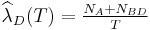${{\widehat{\lambda }}_{D}}(T)=\tfrac{{{N}_{A}}+{{N}_{BD}}}{T}\,\!$.

Thus: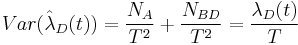$Var({{\hat{\lambda }}_{D}}(t))=\frac{{{N}_{A}}}{{{T}^{2}}}+\frac{{{N}_{BD}}}{{{T}^{2}}}=\frac{{{\lambda }_{D}}(t)}{T}\,\!$

and: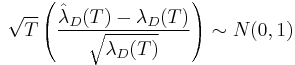$\sqrt{T}\left( \frac{{{{\hat{\lambda }}}_{D}}(T)-{{\lambda }_{D}}(T)}{\sqrt{{{\lambda }_{D}}(T)}} \right)\sim N(0,1)\,\!$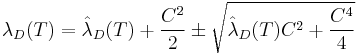${{\lambda }_{D}}(T)={{\hat{\lambda }}_{D}}(T)+\frac{{{C}^{2}}}{2}\pm \sqrt{{{{\hat{\lambda }}}_{D}}(T){{C}^{2}}+\frac{{{C}^{4}}}{4}}\,\!$

where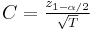$C=\tfrac{{{z}_{1-\alpha /2}}}{\sqrt{T}}\,\!$.

If there are BC failure modes, the demonstrated failure intensity,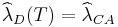${{\widehat{\lambda }}_{D}}(T)={{\widehat{\lambda }}_{CA}}\,\!$, is actually the instantaneous failure intensity based on all of the data.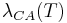${{\lambda }_{CA}}(T)\,\!$ must be positive; thus,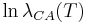$\ln {{\lambda }_{CA}}(T)\,\!$ is approximately treated as being normally distributed.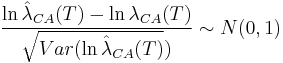$\frac{\ln {{{\hat{\lambda }}}_{CA}}(T)-\ln {{\lambda }_{CA}}(T)}{\sqrt{Var(\ln {{{\hat{\lambda }}}_{CA}}(T)})}\sim N(0,1)\,\!$

The approximate confidence bounds on the instantaneous failure intensity are then estimated from: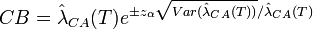$CB={{\hat{\lambda }}_{CA}}(T){{e}^{\pm {{z}_{\alpha }}\sqrt{Var({{{\hat{\lambda }}}_{CA}}(T))}/{{{\hat{\lambda }}}_{CA}}(T)}}\,\!$

where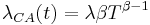${{\lambda }_{CA}}(t)=\lambda \beta {{T}^{\beta -1}}\,\!$.\begin{align} Var({{{\hat{\lambda }}}_{CA}}(T))= & {{\left( \frac{\partial {{\lambda }_{CA}}(T)}{\partial \beta } \right)}^{2}}Var(\hat{\beta })+{{\left( \frac{\partial {{\lambda }_{CA}}(T)}{\partial \lambda } \right)}^{2}}Var(\hat{\lambda }) \\ & +2\left( \frac{\partial {{\lambda }_{CA}}(T)}{\partial \beta } \right)\left( \frac{\partial {{\lambda }_{CA}}(T)}{\partial \lambda } \right)cov(\hat{\beta },\hat{\lambda }) \end{align}\,\!

The variance calculation is the same as described in the Crow-AMSAA Confidence Bounds appendix.

#### Crow Bounds

If there are no BC failure modes then: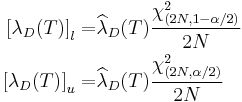\begin{align} {{[{{\lambda }_{D}}(T)]}_{l}}= & {{\widehat{\lambda }}_{D}}(T)\frac{\chi _{(2N,1-\alpha /2)}^{2}}{2N} \\ {{[{{\lambda }_{D}}(T)]}_{u}}= & {{\widehat{\lambda }}_{D}}(T)\frac{\chi _{(2N,\alpha /2)}^{2}}{2N} \end{align}\,\!

where${{\widehat{\lambda }}_{D}}(T)={{\widehat{\lambda }}_{CA}}\,\!$.

If there are BC modes then the confidence bounds on the demonstrated failure intensity are calculated as presented in the Crow-AMSAA Confidence Bounds appendix.

### Bounds on Demonstrated MTBF

#### Fisher Matrix Bounds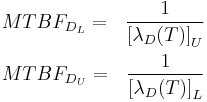\begin{align} & MTB{{F}_{{{D}_{L}}}}= & \frac{1}{{{[{{\lambda }_{D}}(T)]}_{U}}} \\ & MTB{{F}_{{{D}_{U}}}}= & \frac{1}{{{[{{\lambda }_{D}}(T)]}_{L}}} \end{align}\,\!

where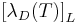${{[{{\lambda }_{D}}(T)]}_{L}}\,\!$ and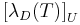${{[{{\lambda }_{D}}(T)]}_{U}}\,\!$ can be obtained from the equation given above for Bounds on Demonstrated Failure Intensity.

#### Crow Bounds\begin{align} MTB{{F}_{{{D}_{L}}}}= & \frac{1}{{{[{{\lambda }_{D}}(T)]}_{U}}} \\ MTB{{F}_{{{D}_{U}}}}= & \frac{1}{{{[{{\lambda }_{D}}(T)]}_{L}}} \end{align}\,\!

where${{[{{\lambda }_{D}}(T)]}_{L}}\,\!$ and${{[{{\lambda }_{D}}(T)]}_{U}}\,\!$ can be obtained from the equation given above for Bounds on Demonstrated Failure Intensity.

### Bounds on Projected Failure Intensity

#### Fisher Matrix Bounds

The projected failure intensity${{\lambda }_{P}}(T)\,\!$ must be positive; thus,$\ln {{\lambda }_{P}}(T)\,\!$ is approximately treated as being normally distributed as well: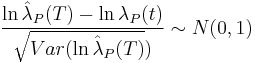$\frac{\ln {{{\hat{\lambda }}}_{P}}(T)-\ln {{\lambda }_{P}}(t)}{\sqrt{Var(\ln {{{\hat{\lambda }}}_{P}}(T)})}\sim N(0,1)\,\!$$CB={{\hat{\lambda }}_{P}}(T){{e}^{\pm {{z}_{\alpha }}\sqrt{Var({{{\hat{\lambda }}}_{P}}(T))}/{{{\hat{\lambda }}}_{P}}(T)}}\,\!$

where:

•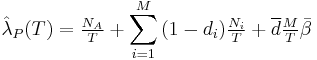${{\hat{\lambda }}_{P}}(T)=\tfrac{{{N}_{A}}}{T}+\underset{i=1}{\overset{M}{\mathop{\sum }}}\,(1-{{d}_{i}})\tfrac{{{N}_{i}}}{T}+\overline{d}\tfrac{M}{T}\bar{\beta }\,\!$ when there are no BC modes.
•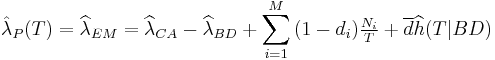${{\hat{\lambda }}_{P}}(T)={{\widehat{\lambda }}_{EM}}={{\widehat{\lambda }}_{CA}}-{{\widehat{\lambda }}_{BD}}+\underset{i=1}{\overset{M}{\mathop{\sum }}}\,(1-{{d}_{i}})\tfrac{{{N}_{i}}}{T}+\overline{d}\widehat{h}(T|BD)\,\!$ when there are BC modes.
•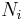${{N}_{i}}\,\!$ is the total failure number of the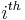${{i}^{th}}\,\!$ distinct BD mode.

You can then get: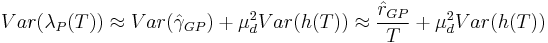$Var({{\lambda }_{P}}(T))\approx Var({{\hat{\gamma }}_{GP}})+\mu _{d}^{2}Var(h(T))\approx \frac{{{{\hat{r}}}_{GP}}}{T}+\mu _{d}^{2}Var(h(T))\,\!$

where: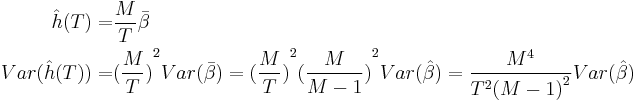\begin{align} \hat{h}(T)= & \frac{M}{T}\bar{\beta } \\ Var(\hat{h}(T))= & {{(\frac{M}{T})}^{2}}Var(\bar{\beta })={{(\frac{M}{T})}^{2}}{{(\frac{M}{M-1})}^{2}}Var(\hat{\beta })=\frac{{{M}^{4}}}{{{T}^{2}}{{(M-1)}^{2}}}Var(\hat{\beta }) \end{align}\,\!

The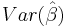$Var(\hat{\beta })\,\!$ can be obtained from Fisher Matrix based on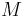$M\,\!$ distinct BD modes.

#### Crow Bounds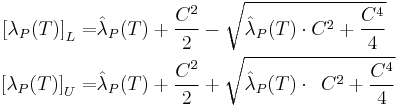\begin{align} {{[{{\lambda }_{P}}(T)]}_{L}}= & {{{\hat{\lambda }}}_{P}}(T)+\frac{{{C}^{2}}}{2}-\sqrt{{{{\hat{\lambda }}}_{P}}(T)\cdot {{C}^{2}}+\frac{{{C}^{4}}}{4}} \\ {{[{{\lambda }_{P}}(T)]}_{U}}= & {{{\hat{\lambda }}}_{P}}(T)+\frac{{{C}^{2}}}{2}+\sqrt{{{{\hat{\lambda }}}_{P}}(T)\cdot \ \,{{C}^{2}}+\frac{{{C}^{4}}}{4}} \end{align}\,\!

where$C=\tfrac{{{z}_{1-\alpha /2}}}{\sqrt{T}}\,\!$.

### Bounds on Projected MTBF

#### Fisher Matrix Bounds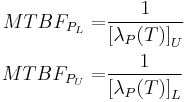\begin{align} MTB{{F}_{{{P}_{L}}}}= & \frac{1}{{{[{{\lambda }_{P}}(T)]}_{U}}} \\ MTB{{F}_{{{P}_{U}}}}= & \frac{1}{{{[{{\lambda }_{P}}(T)]}_{L}}} \end{align}\,\!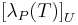${{[{{\lambda }_{P}}(T)]}_{U}}\,\!$ and${{[{{\lambda }_{P}}(T)]}_{L}}\,\!$ can be obtained from the equation given above for Bounds on Projected Failure Intensity.

#### Crow Bounds\begin{align} MTB{{F}_{{{P}_{L}}}}= & \frac{1}{{{[{{\lambda }_{P}}(T)]}_{U}}} \\ MTB{{F}_{{{P}_{U}}}}= & \frac{1}{{{[{{\lambda }_{P}}(T)]}_{L}}} \end{align}\,\!${{[{{\lambda }_{P}}(T)]}_{U}}\,\!$ and${{[{{\lambda }_{P}}(T)]}_{L}}\,\!$ can be obtained from the equation given above for Bounds on Projected Failure Intensity.

### Bounds on Growth Potential Failure Intensity

#### Fisher Matrix Bounds

If there are no BC failure modes, the growth potential failure intensity is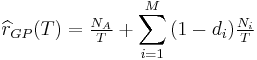${{\widehat{r}}_{GP}}(T)=\tfrac{{{N}_{A}}}{T}+\underset{i=1}{\overset{M}{\mathop{\sum }}}\,(1-{{d}_{i}})\tfrac{{{N}_{i}}}{T}\,\!$.

Then: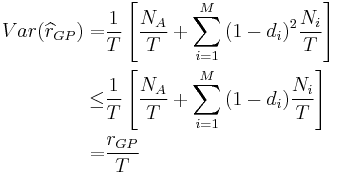\begin{align} Var({{\widehat{r}}_{GP}})= & \frac{1}{T}\left[ \frac{{{N}_{A}}}{T}+\underset{i=1}{\overset{M}{\mathop \sum }}\,{{(1-{{d}_{i}})}^{2}}\frac{{{N}_{i}}}{T} \right] \\ \le & \frac{1}{T}\left[ \frac{{{N}_{A}}}{T}+\underset{i=1}{\overset{M}{\mathop \sum }}\,(1-{{d}_{i}})\frac{{{N}_{i}}}{T} \right] \\ = & \frac{{{r}_{GP}}}{T} \end{align}\,\!

If there are BC failure modes, the growth potential failure intensity is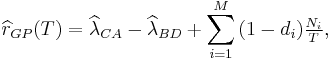${{\widehat{r}}_{GP}}(T)={{\widehat{\lambda }}_{CA}}-{{\widehat{\lambda }}_{BD}}+\underset{i=1}{\overset{M}{\mathop{\sum }}}\,(1-{{d}_{i}})\tfrac{{{N}_{i}}}{T},\,\!$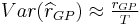$Var({{\widehat{r}}_{GP}})\approx \tfrac{{{r}_{GP}}}{T}\,\!$.

Therefore: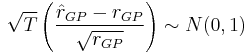$\sqrt{T}\left( \frac{{{{\hat{r}}}_{GP}}-{{r}_{GP}}}{\sqrt{{{r}_{GP}}}} \right)\sim N(0,1)\,\!$

The confidence bounds on the growth potential failure intensity are as follows: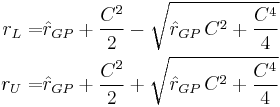\begin{align} {{r}_{L}}= & {{{\hat{r}}}_{GP}}+\frac{{{C}^{2}}}{2}-\sqrt{{{{\hat{r}}}_{GP}}\,{{C}^{2}}+\frac{{{C}^{4}}}{4}} \\ {{r}_{U}}= & {{{\hat{r}}}_{GP}}+\frac{{{C}^{2}}}{2}+\sqrt{{{{\hat{r}}}_{GP}}\,{{C}^{2}}+\frac{{{C}^{4}}}{4}} \end{align}\,\!

where$C=\tfrac{{{z}_{1-\alpha /2}}}{\sqrt{T}}\,\!$.

#### Crow Bounds

The Crow bounds for the growth potential failure intensity are the same as the Fisher Matrix bounds.

### Bounds on Growth Potential MTBF

#### Fisher Matrix Bounds\begin{align} MTB{{F}_{G{{P}_{L}}}}= & \frac{1}{{{r}_{U}}} \\ MTB{{F}_{G{{P}_{U}}}}= & \frac{1}{{{r}_{L}}} \end{align}\,\!

where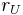${{r}_{U}}\,\!$ and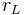${{r}_{L}}\,\!$ can be obtained from the equation given above for Bounds on Growth Potential Failure Intensity.

#### Crow Bounds

The Crow bounds for the growth potential MTBF are the same as the Fisher Matrix bounds.